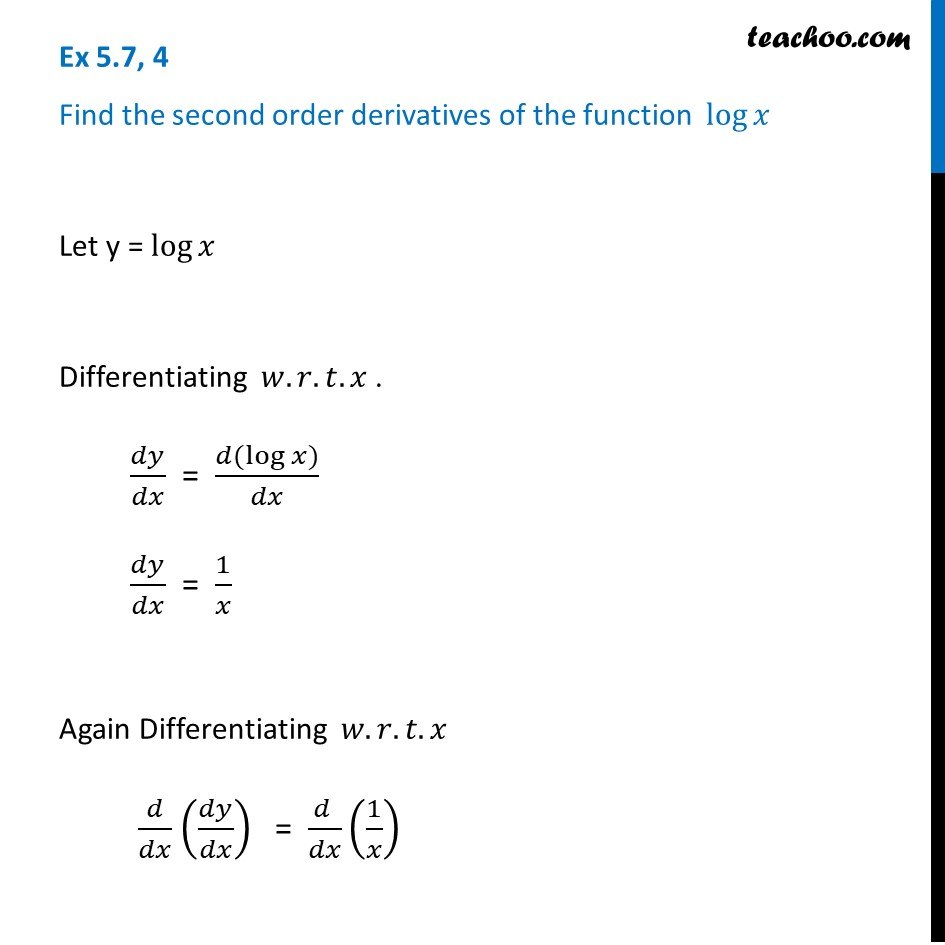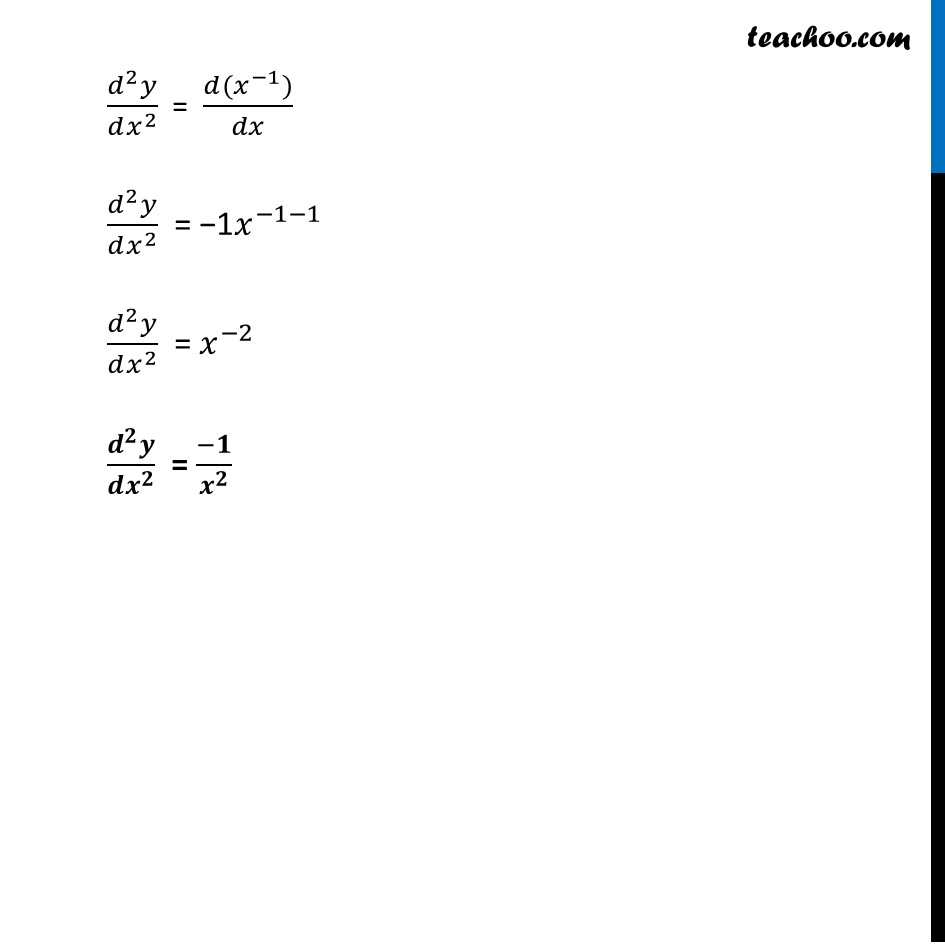Finding second order derivatives - Normal form

Chapter 5 Class 12 Continuity and Differentiability
Concept wiseIntroducing your new favourite teacher - Teachoo Black, at only ₹83 per month

### Transcript

Ex 5.7, 4 Find the second order derivatives of the function log⁡𝑥 Let y = log⁡𝑥 Differentiating 𝑤.𝑟.𝑡.𝑥 . 𝑑𝑦/𝑑𝑥 = (𝑑(log⁡𝑥))/𝑑𝑥 𝑑𝑦/𝑑𝑥 = 1/𝑥 Again Differentiating 𝑤.𝑟.𝑡.𝑥 𝑑/𝑑𝑥 (𝑑𝑦/𝑑𝑥) = (𝑑 )/𝑑𝑥 (1/𝑥) (𝑑^2 𝑦)/(𝑑𝑥^2 ) = (𝑑(𝑥^(−1)))/𝑑𝑥 (𝑑^2 𝑦)/(𝑑𝑥^2 ) = "−1" 𝑥^(−1−1) (𝑑^2 𝑦)/(𝑑𝑥^2 ) = 𝑥^(−2) (𝒅^𝟐 𝒚)/(𝒅𝒙^𝟐 ) = (−𝟏)/𝒙^𝟐